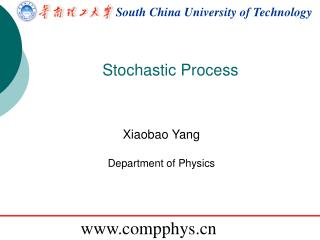DownloadDownload PresentationSouth China University of Technology

# South China University of Technology

Download Presentation## South China University of Technology

- - - - - - - - - - - - - - - - - - - - - - - - - - - E N D - - - - - - - - - - - - - - - - - - - - - - - - - - -
##### Presentation Transcript

1. South China University of Technology Stochastic Process Xiaobao Yang Department of Physics www.compphys.cn

2. Generation of random number

3. circumference ratio

4. Generation Non-uniform random number The possibilities of events A and B is 2:1. The possibilities of events A1, A2 … An is R1, R2 … Rn . The possibilities of events A1, A2 … An is f(1), f(2) … f(n) .

5. Weibull distribution

6. Non-uniform cases uniform distribution x=rand(1000,1); y=-log(x); hist(y)

7. Non-uniform cases (0, Py(max)) If Py(y1) < P test, we remove y1 from the sequence; otherwise it is kept. for ii=1:length(y) if exp(-y(ii))>rand x=[x y(ii)]; end end y=rand(1000,1)*6; x=[];

8. Spatial correlation

9. 帕斯卡 惠更斯

10. Simulation of gambling The big bet induces chaos.

11. SixGL.m Simulation of HK Six Marks There are Events 1~12. You win 10 when you hit it. for ii=2:N banker=floor(rand*12)+1; tmp=floor(rand(10,1)*12)+1; buyers(:,ii)=buyers(:,ii-1)-1+10*(tmp==banker); end

13. Summary • Select a proper distribution • Construct the Stochastic Process • Store the data for histogram.

14. The existence of god of gamblers? 1 1 1 0 0 1 0 0 1 0 …. When you bet N times, the possibility of all win is 2^(-N). Don't get crush on him and he is just a legend.

15. Random walks

16. Random walks • Concept of random walk. x0=0 • Informative quantity:

17. 1-D random walks • clear • N=300; • M=100; • re=zeros(M,N); • for ii=1:N-1 • for jj=1:M • re(jj,ii+1)=re(jj,ii)+sign(rand-0.5); • end • end • plot(sum(re.^2,1)/M) rw1d.m

18. Random walks • Questions: To make it more realistic: • What is the relation of <r2> vs. t for a random walk in 2-D, 3-D systems? • How about allow the steps to be a random length in [-1,1]?

19. 2-D random walks • clear • N=300; • M=100; • re=zeros(M*2,N); • for ii=1:N-1 • for jj=1:M • if rand <0.5 • re(jj*2-1,ii+1)=re(jj*2-1,ii)+sign(rand-0.5); • re(jj*2,ii+1)=re(jj*2,ii); • else • re(jj*2,ii+1)=re(jj*2,ii)+sign(rand-0.5); • re(jj*2-1,ii+1)=re(jj*2-1,ii); • end • end • end • plot(sum(re.^2,1)/M) rw2d.m

20. Random walk in hexagonal lattice O (0.00,0.00) A (0.00,1.00) B (-0.87 1.50) C ( 0.87 1.50) T=

21. Self-Avoiding Walks A typical polymer in solution may be described by SAW. Keep all possible SAW configurations equivalent. To generate 4th steps if a new possible way is chosen when “self-avoiding” • There are 3 possible ways. (only has 2/3 the probability as in b) • There are only 2 possible ways . How to correct the problem? • Discard the whole RW when restriction condition occurred. CPU time ! • “enumeration” instead of “simulation”.

22. saw.m jj=1:sx(1) newtmp=[x(jj,1:2:end)' x(jj,2:2:end)']; % find all the used sites in the same link! tmp=x(jj,end-1:end)+[0 1]; if length(intersect(tmp,newtmp,'rows'))==0; allx=[allx x(jj,:) tmp]; end

23. Self-Avoiding Walks Log-log scale Average of 106 walks Average of 1000 walks ν=1/2: simple random walk ν=3/4: 2D SAW ν~3/5: 3D SAW

24. Walker with memory 可以且仅可以记忆它上一步走过的方向并避免重复， 比如当从(0,0)走到(0,1)时，下一步只有3个可能的位移[(0,2), (1,1), (-1,1)]， 避免了从(0,1)走回(0,0)位置。 while (re(jj*2-1,ii+1)==re(jj*2-1,ii-1)&re(jj*2,ii+1)==re(jj*2,ii-1)) if rand <0.5 re(jj*2-1,ii+1)=re(jj*2-1,ii)+sign(rand-0.5); re(jj*2,ii+1)=re(jj*2,ii); else re(jj*2,ii+1)=re(jj*2,ii)+sign(rand-0.5); re(jj*2-1,ii+1)=re(jj*2-1,ii); end walkwith.m

25. Homework Random walk in triangular or hexagonal lattice. Sending to 17273799@qq.com when ready For lecture notes, refer to http://www.compphys.cn/~xbyang/